# Subtract Numbers in Excel Using Formula and Functions

In this tutorial, learn how to subtract numbers in Excel using formula and functions. You can subtract two or more numbers in excel using the useful methods given here.

You have to use the minus(-) operator to subtract two numbers. However, there is no function in excel to perform this task. Find out methods with screenshot given in each step for more help.

## Subtract Two Numbers in Excel Using Minus(-) Operator

1. Enter Two Numbers for Subtraction
Put any two numbers in the cell which you want to find the subtraction. You have to use these numbers to calculate the difference between these two numbers.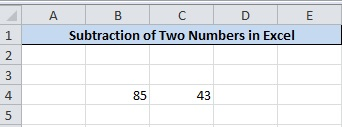The above image showing the two number which you want to get the difference as the output. The cell is B4 and C4 with B4 contains the largest number and C4 contains the smallest number.

2. Place equals to(=) operator Where you have to Calculate Subtraction
Now, suppose you have to calculate the difference between these two numbers in the cell D4. You have to place the equals to(=) operator in the cell D4 to start the formula in Excel.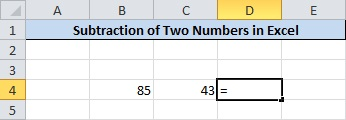Check the above image contains the equals to(=) operator as the starting point of the calculation.

3. Press ‘CTRL’ and Select Two Numbers By Clicking on it
Press the ‘CTRL’ button and hold it and mouse click the two numbers. On click of the cell, the name of the cell automatically gets inserted. Don’t forget to place the minus(-) sign between the numbers.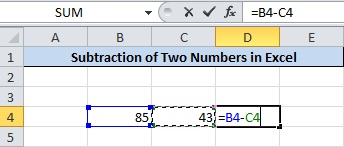The above image showing the completed formula of subtraction which is read to give the output.

4. Final Step is to Press Enter For the Final Result
This is the final step of this method where you have to press the ‘Enter’ button of the keyboard. The calculated result starts showing in the cell D4.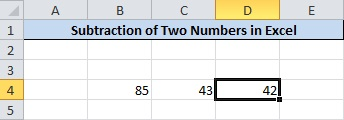## How To Find Difference of More Than Two Numbers in Excel

If there are more than two numbers for which you have to calculate the subtraction. You have to follow the below given step-by-step process.

1. Enter More Than Two Numbers
Let’s take 17, 5, 7, 9 and 3 as the five numbers you have to calculate the difference. Place these numbers in a single column of the cell sequentially.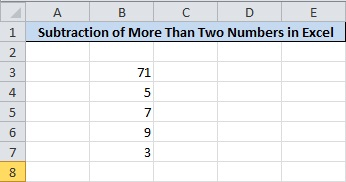The numbers are showing in the image above from cell B3 to B7.

2. Find the Place to Calculate Subtraction and Put equals to(=) Operator
Now, suppose you have to calculate the subtraction of these numbers in the cell D5. You have to place the equals to(=) operator to start the calculation.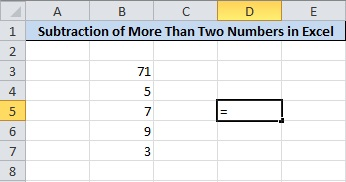The equals to(=) operator is required to start the formula for any calculation in the Excel.

3. Press ‘CTRL’ and Select Cell Followed By minus(-) Sign
Now select all the cells contains the numbers in the Excel sheet. Pressing and hold the ‘CTRL’ key and mouse click each number from top to bottom. Also, add the minus(-) sign between every two numbers as showing in the image below.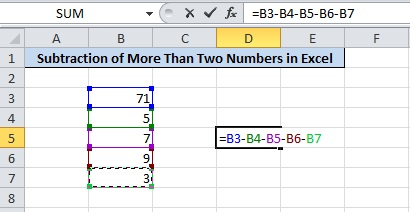The above images showing the selected numbers and the numbers printed automatically. Don’t forget to place the minus(-) sign after every two numbers as shown above.

4. Press ‘Enter’ For Final Result
You just have to press the ‘Enter’ button and you are all done. The output starts displaying in the cell D5.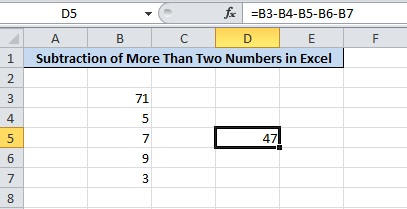47 is the difference between these five numbers in the Excel sheet shown above in the image.

## Calculate Difference Between Two Columns in Excel

1. Enter numbers in Two Columns to Subtract
Place all the numbers in the Col1 and Col2. There are five rows of numbers to calculate the difference.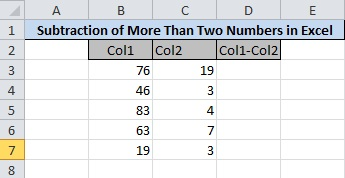2. Place equals (=) Operator in The First Rows of Data Under (Col1-Col2) Cell
Let’s start calculating the difference from the first row after heading Col1 and Col2. The cell B3 contains the number 76 and C3 contains the number 19. Place equals to(=) operator in the cell after the heading Col1-Col2.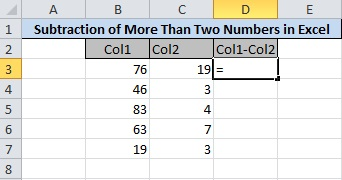3. Press ‘CTRL’ and Select Col1 and Col2 Cells Followed By Minus(-)
Select the two numbers to calculate the difference. Press and hold ‘CTRL’ key and click the cell B3 and C3. Don’t forget to place the minus(-) sign between the numbers.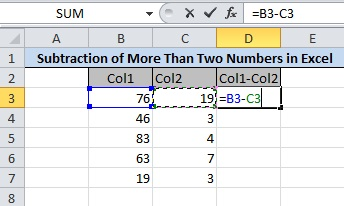4. Press Enter to Get the Subtraction in the First Row
Now, press the enter button to get the final result in the output. The below image showing the number 57 as the difference between the first two numbers.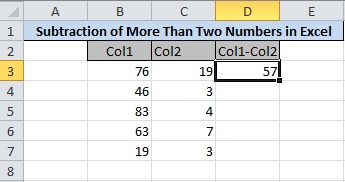5. Click Indicated Place and Hold the Mouse Left Button and Drag Mouse Till the Last Row
To calculate the difference of other numbers given in the next rows. You have to click and hold the mouse right button. Drag down till you reach the last row contains the numbers.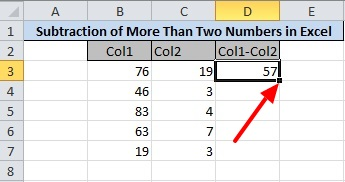6. Final Result of Two Column Subtraction
You will get the calculation of differences of all other rows numbers. Check the final result you will in the image given below.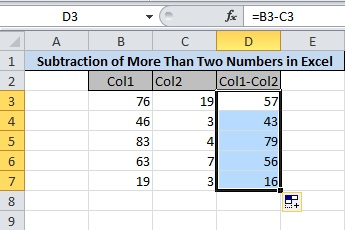You may also like to read

Hope, you like this post of how to subtract numbers in Excel. If you have any query regarding the tutorial, please comment below.

Also tell me, which method you are using to calculate the difference of numbers in Excel.## THE ULTIMATE TOOLKIT

Get FREE Access to Toolkit and Resources that we are using in Tutorialdeep that Every Professional Should Have! in one handy PDF.